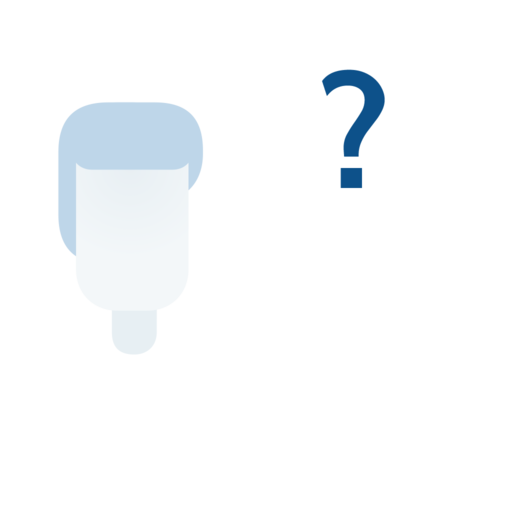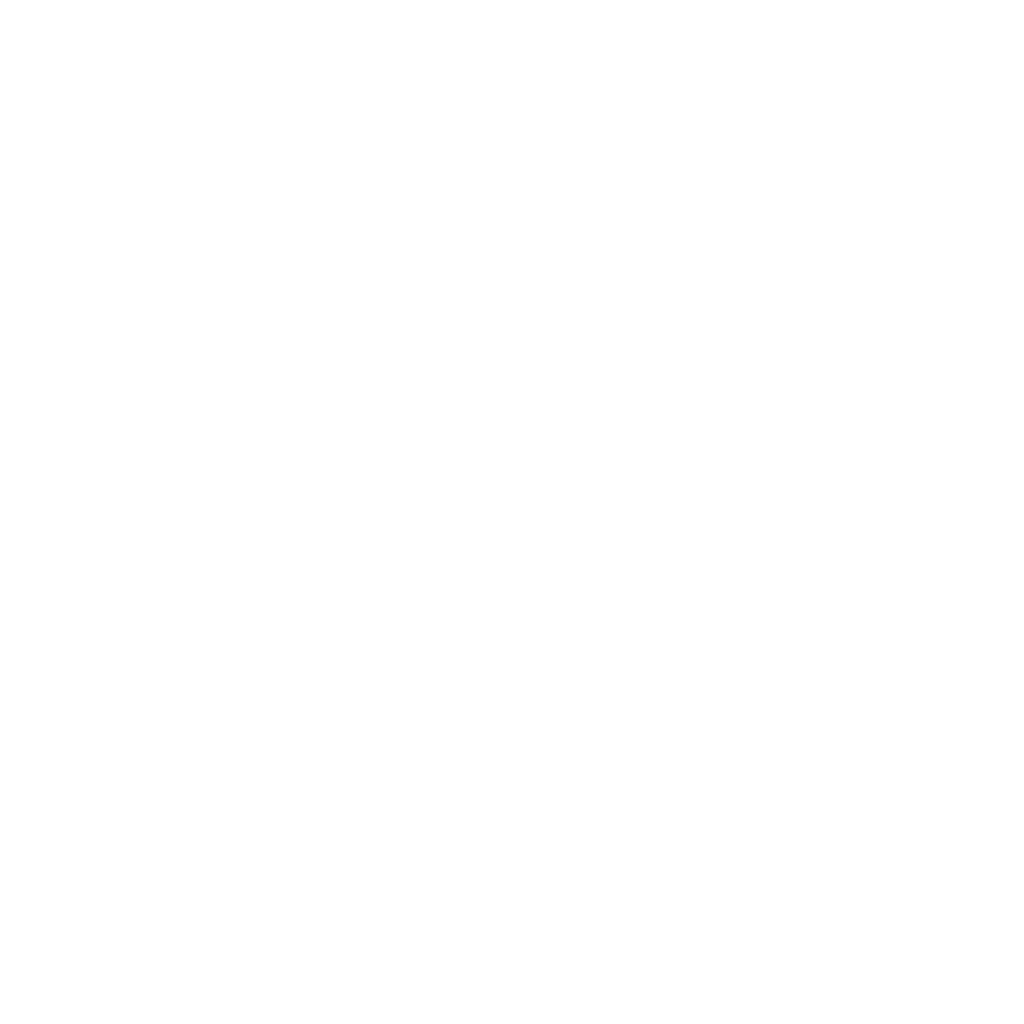## Pronomi e Aggettivi Interrogativi

Introduction Introduzione
There are two ways of asking questions in Italian. The first is by simply adding a rising intonation or question mark to the end of a statement. Making questions this way require no change in word order as with some other languages:

Tu balli.
You dance.

Tu balli?
Do you dance?

The second way is by using interrogative words. These are the English equivalents of who, what, when, where, which, why, how and how many. The interrogatives can be divided into two categories: pronouns and adjectives like “what” and “which”, which replace or modify nouns, and adverbs like "when" and "how", which modify a verb. In this lesson, you will learn the interrogative pronouns and adjectives.

The main difference in how Italian uses interrogative pronouns/adjectives is that it has two forms of “which” (quale and quali) to ask about singular and plural objects, and four form of “how many/how much” (quanto, quanta, quanti and quante) which change for singular and plural objects and based on gender.
Masculine Femininequanto how manyquanta how manyquanti how manyquante how many
Change languageFrançaisEspañolEnglishItalianoDeutschPortuguês